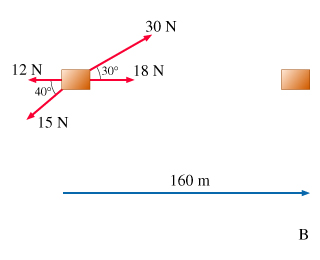# Problem: Find the work W done by the 12-newton force and the 15-newton force

###### FREE Expert Solution

Work done:

The angle between two parallel vectors is 0 while the angle between antiparallel vectors is 180.

The angle between the 12 N force and the direction of motion is 180°.

100% (411 ratings)###### Problem DetailsFind the work W done by the 12-newton force and the 15-newton force